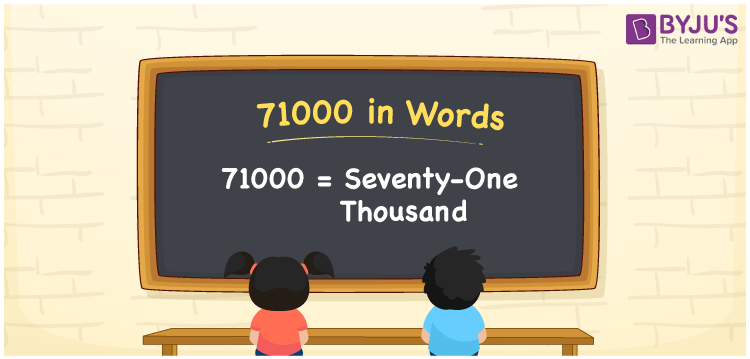# 71000 in words

71000 in words is written as Seventy One Thousand. In 71000, 7 has a place value of ten thousand and 1 has a place value of thousand. The article on Place Value gives more information. The number 71000 is used in expressions that relate to money, distance, length, social media view, and many more. For example, “Seventy One Thousand is the number of subscribers to a newly started Youtube channel.” “There were Seventy One Thousand orders for newly launched smartphones on day 1.”

 71000 in words Seventy One Thousand Seventy One Thousand in Numbers 71000

## 71000 in English Words## How to Write 71000 in Words?

We can convert 71000 to words using a place value chart. The number 71000 has 5 digits, so let’s make a chart that shows the place value up to 5 digits.

 Ten thousand Thousands Hundreds Tens Ones 7 1 0 0 0

Thus, we can write the expanded form as:

7 × Ten thousand + 1 × Thousand + 0 × Hundred + 0 × Ten + 0 × One

= 7 × 10000 + 1 × 1000 + 0 × 100 + 0 × 10 + 0 × 1

= 71000

= Seventy One Thousand.

71000 is the natural number that is succeeded by 70999 and preceded by 71001.

71000 in words – Seventy One Thousand.

Is 71000 an odd number? – No.

Is 71000 an even number? – Yes.

Is 71000 a perfect square number? – No.

Is 71000 a perfect cube number? – No.

Is 71000 a prime number? – No.

Is 71000 a composite number? – Yes.

## Solved Example

1. Write the number 71000 in expanded form

Solution: 7 x 10000 + 1 x 1000 + 0 x 100 + 0 x 10 + 0 x 1

Or Just 7 x 10000 + 1 x 1000

We can write 71000 = 70000 + 1000 + 0 + 0 + 0

= 7 x 10000 + 1 x 1000 + 0 x 100 + 0 x1 0 + 0 x 1

## Frequently Asked Questions on 71000 in words

Q1

### How to write 71000 in words?

71000 in words is written as Seventy One Thousand.
Q2

### State whether True or False. 71000 is divisible by 3?

False. 71000 is not divisible by 3.
Q3

### Is 71000 divisible by 10?

Yes. 71000 is divisible by 10.